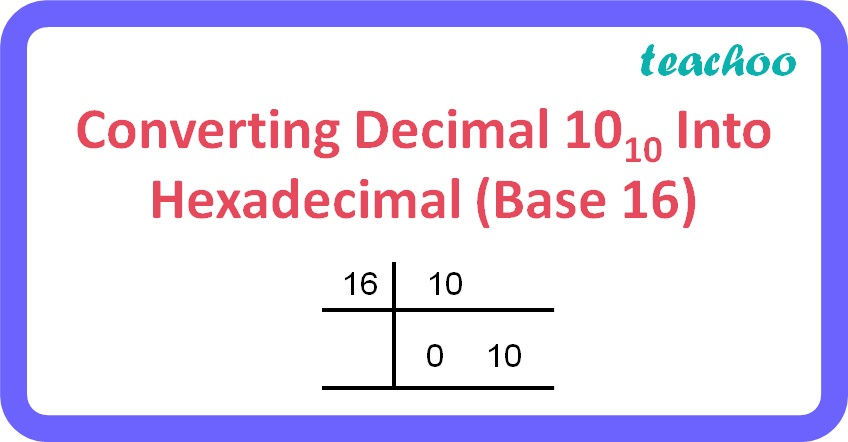MCQ questions (1 mark each)

Computer Science - Class 11
Chapter 2 Class 11 - Encoding Schemes and Number System

## (d) F 16

10 10 is a decimal number.

To find the hexadecimal equivalent of a decimal number:

• Repeatedly divide the decimal number by 16 until the quotient becomes less than 16 and record all the remainders.
• The remainders should be written bottom to upwards to get the hexadecimal equivalent of the decimal number.

Here,

• 10 = A
• 11 = B
• 12 = C
• 13 = D
• 14 = E
• 15 = FRemainder = 010 = 10

Given, 10 = A

Therefore, 10 10 = A 16

So, the correct answer is (c).

Get live Maths 1-on-1 Classs - Class 6 to 12